Question

# A nominal interest rate of 10% per year, compounded monthly, is most closely:   1% per...

A nominal interest rate of 10% per year, compounded monthly, is most closely:

 1% per month
 5.11% per six months
 10% per year
 4.98% per six months

Interest rate 10%

per month interest 0.008333333

compounded montly per year interest rate 1.104713067-1 = 0.104713 = 10.47%

compounded montly six months interest rate 1.051053313 - 1 = 0.051053 = 5.105331 %

So option 2 is the answer 5.11% per six months

#### Earn Coins

Coins can be redeemed for fabulous gifts.

Similar Homework Help Questions
• ### For an APR of 10% per year, if the interest is compounded weekly, determine the nominal...

For an APR of 10% per year, if the interest is compounded weekly, determine the nominal rate per (a) 6 months and (b) 2 years. The nominal interest rate per 6 months is %. The nominal interest rate per 2 years is %.

• ### What effective interest rate per two-months, compounded continuously, is equivalent to a nominal rate of 10%...

What effective interest rate per two-months, compounded continuously, is equivalent to a nominal rate of 10% per year? The effective interest rate per two-months is _______ %

• ### 2. What nominal annual interest rate compounded monthly is equivalent to an effective annual interest rate...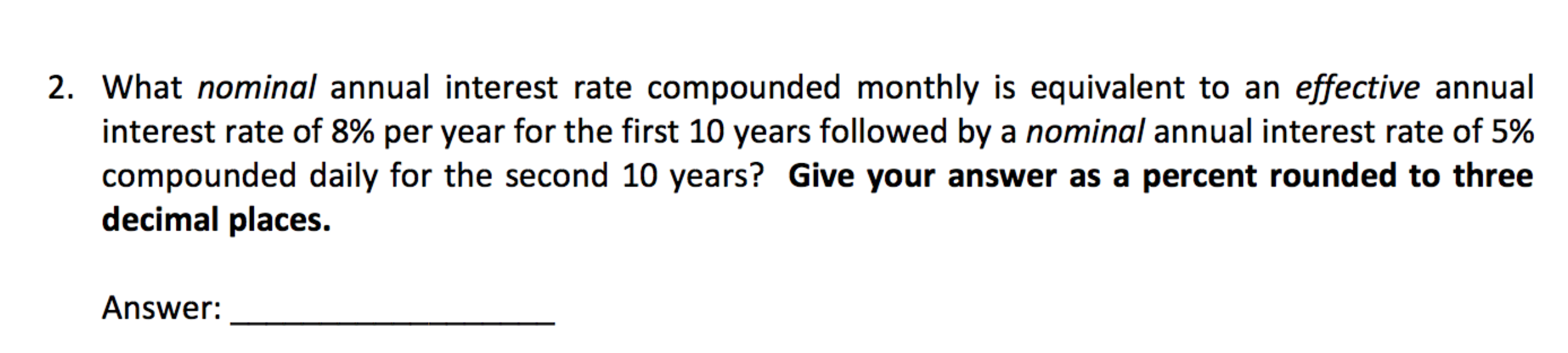2. What nominal annual interest rate compounded monthly is equivalent to an effective annual interest rate of 8% per year for the first 10 years followed by a nominal annual interest rate of 5% compounded daily for the second 10 years? Give your answer as a percent rounded to three decimal places. Answer:

• ### For an APR of 9% per year, if the interest is compounded daily, determine the nominal...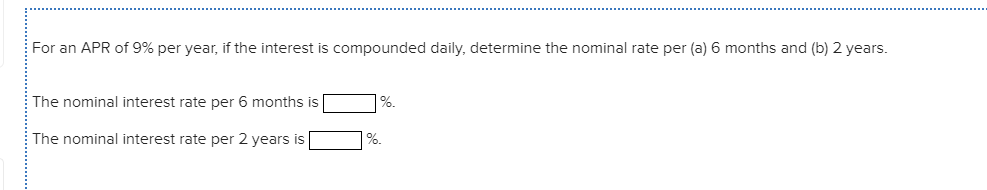For an APR of 9% per year, if the interest is compounded daily, determine the nominal rate per (a) 6 months and (b) 2 years. The nominal interest rate per 6 months is 1%. The nominal interest rate per 2 years is L %.

• ### If the nominal annual interest rate is 24% compounded continuously, then the effective interest rate per...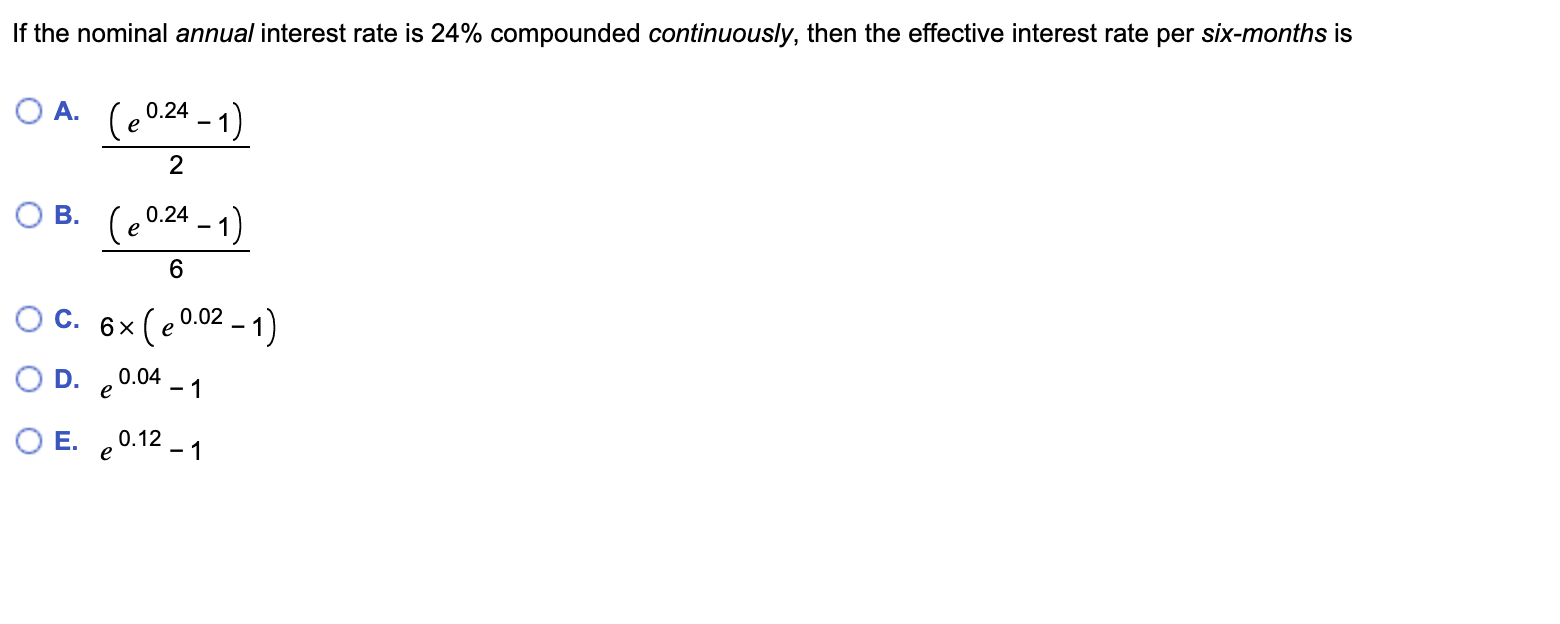If the nominal annual interest rate is 24% compounded continuously, then the effective interest rate per six-months is OA. (20.24 - 1) OB. (20.24 - 1) O C. 6x (20.02 - 1) OD. €0.04 - 1 O E. 20.12 - 1

• ### 1. If the nominal interest rate of is 2% per quarter, what is the nominal rate...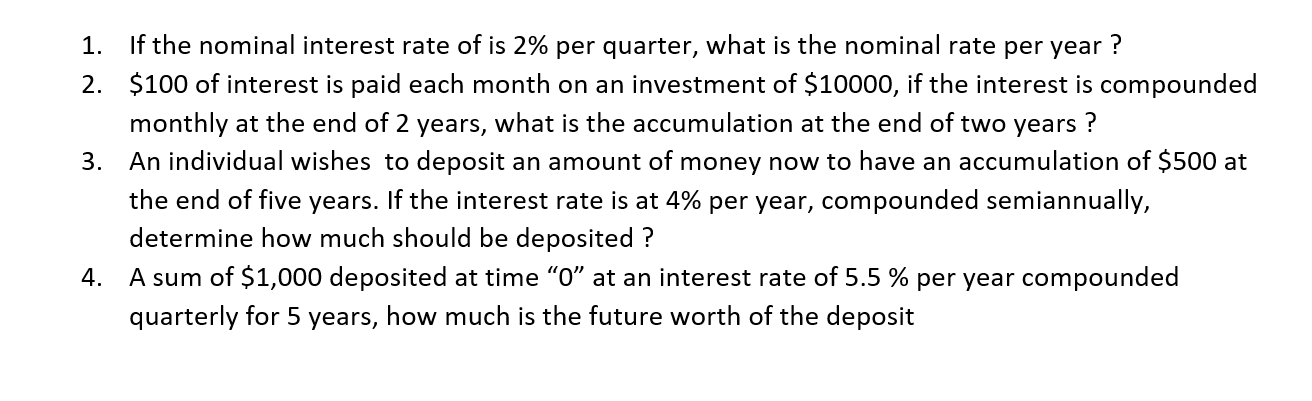1. If the nominal interest rate of is 2% per quarter, what is the nominal rate per year ? 2. \$100 of interest is paid each month on an investment of \$10000, if the interest is compounded monthly at the end of 2 years, what is the accumulation at the end of two years ? 3. An individual wishes to deposit an amount of money now to have an accumulation of \$500 at the end of five years. If the...

• ### An interest rate of 2% per month is the same as: O A) 24% per year...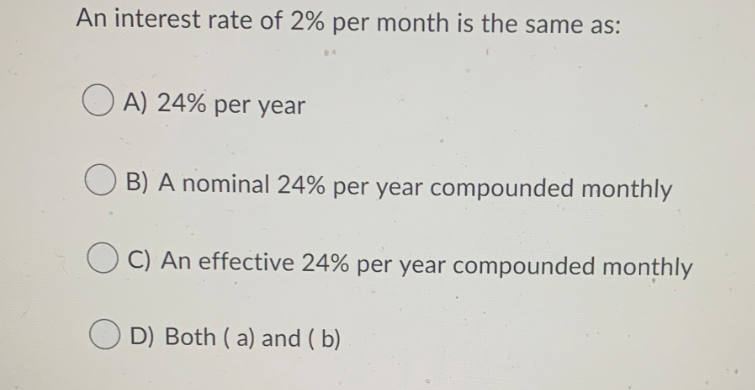An interest rate of 2% per month is the same as: O A) 24% per year B) A nominal 24% per year compounded monthly C) An effective 24% per year compounded monthly OD) Both ( a) and (b) Identify each of the following interest rate statements as either nominal or effective. V 12% per year compounded semiannually 0.1% per day compounded hourly 6% per year compounded annually 1. Nominal 4% per year 2. Effective 8% per year compounded monthly 1%...

• ### 8? 8. An interest rate of 10% per year compounded continuously is the same as: C...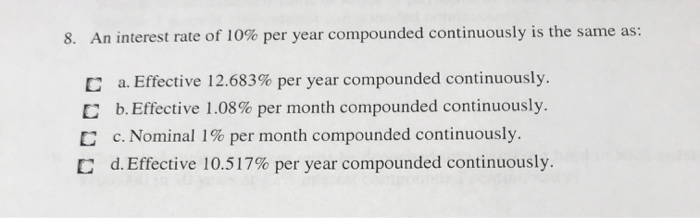8? 8. An interest rate of 10% per year compounded continuously is the same as: C D a. Effective 12.683% per year compounded continuously. b. Effective 1.08% per month compounded continuously. c. Nominal 1% per month compounded continuously. d. Effective 10.517% per year compounded continuously. C

• ### With a nominal annual interest rate of 6.0%, compounded monthly, at the end of 1 year,...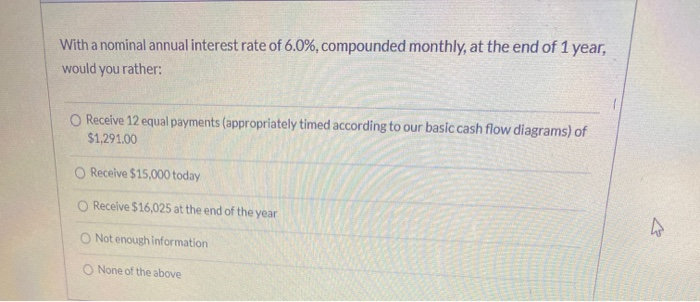With a nominal annual interest rate of 6.0%, compounded monthly, at the end of 1 year, would you rather: Receive 12 equal payments (appropriately timed according to our basic cash flow diagrams) of \$1,291.00 Receive \$15,000 today Receive \$16,025 at the end of the year Not enough information None of the above

• ### 10% per vear compounded monthly this interest rate is a an interest is advertised in the...10% per vear compounded monthly this interest rate is a an interest is advertised in the newspaper as 'APY nominal rate True False Question 2 (4 points) Saved A nominal interest is an interest rate that does not account for compounding. In other words, a nominal Interest rate, is an interest rate that does not include any consideration of compounding True False Question 3 (4 points) Saved rest i ti med to be equal to the period over which the...